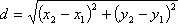• Author: Graham Mitchell
• Filename: DistanceFormula.java

### Distance Formula

Write a function to compute the distance between two points. Given two points (x1, y1) and (x2, y2), the distance between these points is given by the formula:You must name the function `distance`, and it must return a `double` giving the distance between the two points.

#### Files Needed

```
public class DistanceFormula
{
public static void main( String[] args )
{
// test the formula a bit
double d1 = distance(-2,1 , 1,5);
System.out.println(" (-2,1) to (1,5) => " + d1 );

double d2 = distance(-2,-3 , -4,4);
System.out.println(" (-2,-3) to (-4,4) => " + d2 );

System.out.println(" (2,-3) to (-1,-2) => " + distance(2,-3,-1,-2) );

System.out.println(" (4,5) to (4,5) => " + distance(4,5,4,5) );
}

public static double distance( int x1, int y1, int x2, int y2 )
{
// put your code up in here
}
}
```
```
(-2,1) to (1,5) => 5.0
(-2,-3) to (-4,4) => 7.280109889280518
(2,-3) to (-1,-2) => 3.1622776601683795
(4,5) to (4,5) => 0.0
```
```
```